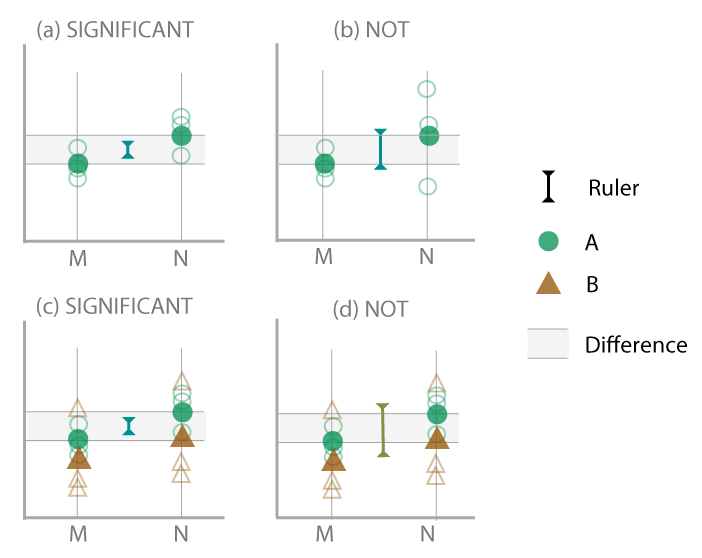Figure 2: Diagram illustrating how estimation of the variance can a_ect the interpretation of the mean di_erence, under di_erent scenarios. (a) Conventional situation, where each condition (M, N) has the same small variance, mean di_erence is viewed as signi_cant, because it is big relative to the small variance. (b) One condition (N) has higher variance than the other, ignoring the di_erence, and pooling the variances would increase the length of the ruler upon which the di_erence is gauged, to the extent that the di_erence is not considered to be signi_cant. (c) Addition of an extra treatment level (B), that has larger variance, but this has no e_ect because each level of the treatments (A, B) is handled as separate samples { di_ erence is still seen as signi_cant. (d) Variances across treatments (A, B) are pooled, which increases the length of the ruler upon which the di_erence is measured, resulting in no signi_cant di_erence.